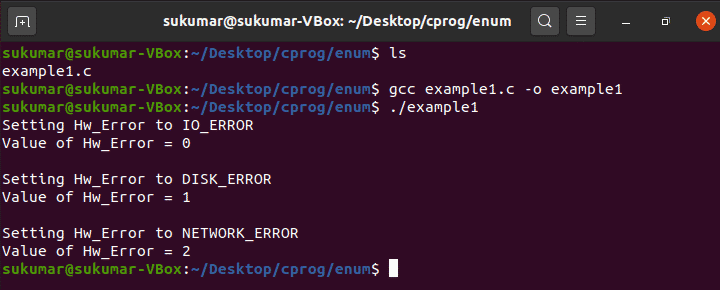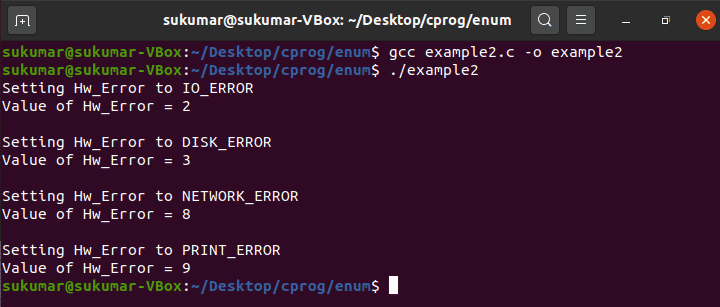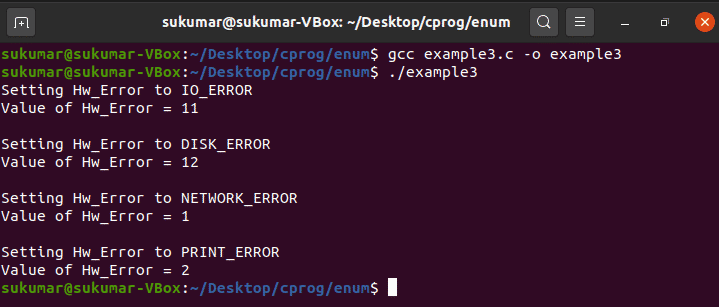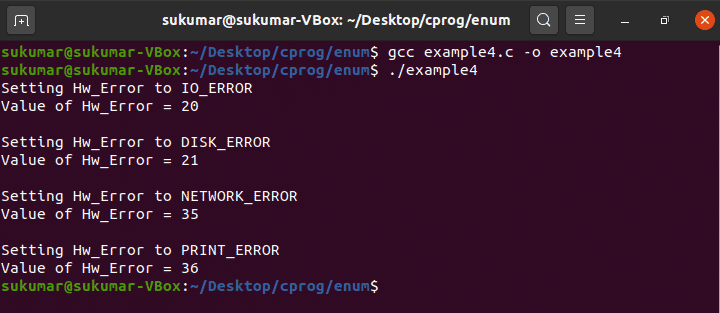C Programming

# How to use enum in C Language

The enum program in the C programming language is used to define integral constant values, which is very helpful in writing clean and readable programs. Programmers normally use enumeration to define named integral constants in their programs to provide better readability and maintainability of the software. This article will discuss enum in detail.

### Syntax

enum  <Enum Type Name> {
Enumeration_Constant_Element-1,
Enumeration_Constant_Element-2,
Enumeration_Constant_Element-3,
…….........,
Enumeration_Constant_Element-n,
};

The default value of Enumeration_Constant_Element-1 is 0, the value of Enumeration_Constant_Element-2 is 1, the value of Enumeration_Constant_Element-3 is 2, and the value of Enumeration_Constant_Element-n is (n-1).

### Deep Dive into Enum

Now, since we know the syntax to define the enumeration type, let us look at an example:

enum Error {
IO_ERROR,
DISK_ERROR,
NETWORK_ERROR
};

The “enum” keyword must always be used to define the enumeration type. So, whenever you want to define an enumeration type, you must use the “enum” keyword before <Enum Type Name>. After the “enum” keyword, you must use a valid identifier to define the <Enum Type Name>.

In the above example, the compiler will assign IO_ERROR to the integral value: 0, DISK_ERROR to the integral value: 1 and NETWORK_ERROR to the integral value: 2.  By default, the first enum-element is always assigned the value 0, the next enum-element is assigned the value 1, and so on.

This default behaviour can be changed if necessary by assigning the constant integral value explicitly, as follows:

enum Error {
IO_ERROR = 2,
DISK_ERROR,
NETWORK_ERROR = 8 ,
PRINT_ERROR
};

In this case, the IO_ERROR is explicitly assigned to a value of 2 by the programmer, DISK_ERROR is assigned to a value of 3 by the compiler, NETWORK_ERROR is explicitly assigned to the value of 8 by the programmer, and PRINT_ERROR is assigned to the next integral value of the previous enum element NETWORK_ERROR (i.e., 9) by the compiler.

So, you now understand how to define a user-defined enumeration type in C. Is it possible to declare a variable of enum type (as we can declare a variable of integer type)?  Yes, it is! You can declare the enum variable as follows:

enum Error Hw_Error;

Again, “enum” is the keyword here, “Error” is the enum type, and  “Hw_Error” is an enum variable.

We will now look at the following examples to understand the various usages of enum:

• Example 1: Default enum definition usage
• Example 2: Custom enum definition usage
• Example 3: enum definition using constant expression
• Example 4: enum scope

### Example 1: Default enum Definition Usage

In this example, you will learn how to define the enumeration type with default constant values. The compiler will take care of assigning the default values to the enum elements. Below, you will see the example program and the corresponding output.

#include <stdio.h>

/* Define the enum type */
enum Error {
IO_ERROR,
DISK_ERROR,
NETWORK_ERROR
};

int main()
{
enum Error Hw_Error;    /* Creating enum variable*/

printf("Setting Hw_Error to IO_ERROR\n");
Hw_Error = IO_ERROR;
printf("Value of Hw_Error = %d \n",Hw_Error);

printf("\nSetting Hw_Error to DISK_ERROR\n");
Hw_Error = DISK_ERROR;
printf("Value of Hw_Error = %d \n",Hw_Error);

printf("\nSetting Hw_Error to NETWORK_ERROR\n");
Hw_Error = NETWORK_ERROR;
printf("Value of Hw_Error = %d \n",Hw_Error);

return 0;
}### Example 2: Custom enum Definition Usage

In this example, you will learn how to define the enumeration type with a custom constant value. Also, this example will help you understand how the custom constants initialization can be done in any random order. In this example, we have explicitly defined the constant value for the 1st and 3rd enum elements (i.e., IO_ERROR and NETWORK_ERROR, respectively), but we have skipped the explicit initialization for the 2nd and 4th elements. It is now the responsibility of the compiler to assign the default values to the 2nd and 4th enum elements (i.e., DISK_ERROR and PRINT_ERROR, respectively). DISK_ERROR will be assigned to a value of 3 and PRINT_ERROR will be assigned to a value of 9. Below, you will see the example program and the output.

#include <stdio.h>

/* Define the enum type  - Custom initialization*/
enum Error {
IO_ERROR = 2,
DISK_ERROR,
NETWORK_ERROR = 8,
PRINT_ERROR
};

int main()
{

/* Declare enum variable*/
enum Error Hw_Error;

printf("Setting Hw_Error to IO_ERROR\n");
Hw_Error = IO_ERROR;
printf("Value of Hw_Error = %d \n",Hw_Error);

printf("\nSetting Hw_Error to DISK_ERROR\n");
Hw_Error = DISK_ERROR;
printf("Value of Hw_Error = %d \n",Hw_Error);

printf("\nSetting Hw_Error to NETWORK_ERROR\n");
Hw_Error = NETWORK_ERROR;
printf("Value of Hw_Error = %d \n",Hw_Error);

printf("\nSetting Hw_Error to PRINT_ERROR\n");
Hw_Error = PRINT_ERROR;
printf("Value of Hw_Error = %d \n",Hw_Error);

return 0;
}### Example 3: Enum Definition Using Constant Expression

In this example, you will learn how to use the constant expression to define the constant value for enum elements.

#include <stdio.h>

/* Define the enum type - custom initialization using constant expression
constant expression is being used here in case of :
a. IO_ERROR and
b. NETWORK_ERROR
This is an unusual way of defining the enum elements; however, this
program demonstrates that this way of enum elements initialization is possible in c.
*/

enum Error {
IO_ERROR = 1 + 2 * 3 + 4,
DISK_ERROR,
NETWORK_ERROR = 2 == 2,
PRINT_ERROR
};

int main()
{

/* Declare enum variable*/
enum Error Hw_Error;

printf("Setting Hw_Error to IO_ERROR\n");
Hw_Error = IO_ERROR;
printf("Value of Hw_Error = %d \n",Hw_Error);

printf("\nSetting Hw_Error to DISK_ERROR\n");
Hw_Error = DISK_ERROR;
printf("Value of Hw_Error = %d \n",Hw_Error);

printf("\nSetting Hw_Error to NETWORK_ERROR\n");
Hw_Error = NETWORK_ERROR;
printf("Value of Hw_Error = %d \n",Hw_Error);

printf("\nSetting Hw_Error to PRINT_ERROR\n");
Hw_Error = PRINT_ERROR;
printf("Value of Hw_Error = %d \n",Hw_Error);

return 0;
}### Example 4: enum Scope

In this example, you will learn how the scoping rule works for enum. A MACRO (#define) could have been used to define a constant instead of the enum, but the scoping rule does not work for MACRO.

#include <stdio.h>

int main()
{

/* Define the enum type */
enum Error_1 {
IO_ERROR = 10,
DISK_ERROR,
NETWORK_ERROR = 3,
PRINT_ERROR
};

{

/* Define the enum type in the inner scope*/
enum Error_1 {
IO_ERROR = 20,
DISK_ERROR,
NETWORK_ERROR = 35,
PRINT_ERROR
};

/* Declare enum variable*/
enum Error_1 Hw_Error;
printf("Setting Hw_Error to IO_ERROR\n");
Hw_Error = IO_ERROR;
printf("Value of Hw_Error = %d \n",Hw_Error);

printf("\nSetting Hw_Error to DISK_ERROR\n");
Hw_Error = DISK_ERROR;
printf("Value of Hw_Error = %d \n",Hw_Error);

printf("\nSetting Hw_Error to NETWORK_ERROR\n");
Hw_Error = NETWORK_ERROR;
printf("Value of Hw_Error = %d \n",Hw_Error);

printf("\nSetting Hw_Error to PRINT_ERROR\n");
Hw_Error = PRINT_ERROR;
printf("Value of Hw_Error = %d \n",Hw_Error);
}
return 0;
}### Comparison Between enum and macro

 Enum Macro Scoping rule is applicable for enum. Scoping rule is not applicable for Macro. Default Enum value assignment happens automatically. Enum is very helpful in defining a large number of constants. The compiler takes the default constant value initialization. The macro constant values must always be mentioned explicitly by the programmer. This could be a tedious process for a large number of constants since the programmer must always manually define each constant value while defining the Macro.

### Conclusion

The enum program in C could be considered an optional method for standalone programs or small-sized projects since programmers can always use macro instead of an enum. However, experienced programmers tend to use enum over macro for large-scale software development projects. This helps in writing clean and readable programs.